# capi/touch.c File Reference

`#include "touch.h"`
`#include <type.h>`
`#include <lpc2xxx.h>`
Include dependency graph for touch.c: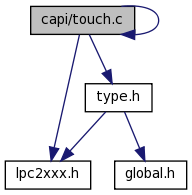This graph shows which files directly or indirectly include this file: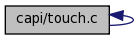## Defines

#define CHX   0x90
#define CHY   0xd0
#define LOSS_DATA   5

## Functions

void SetTouch_DIN (UINT8 value)
void start_touch ()
void TP_Init ()
void TP_Done ()
UINT8 TP_GetAdXYOm (UINT16 *x, UINT16 *y)

## Define Documentation

 #define CHX   0x90

 #define CHY   0xd0

 #define LOSS_DATA   5

## Function Documentation

 UINT16 Read_XY ( UINT8 xy )

Here is the call graph for this function: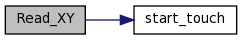void SetTouch_DIN ( UINT8 value )

References TP_DIN_H, and TP_DIN_L.

 void start_touch ( )

References TP_CS_H, TP_CS_L, TP_DCLK_H, TP_DCLK_L, and TP_DIN_H.

Here is the caller graph for this function:References TP_DCLK_H, and TP_DCLK_L.

Here is the caller graph for this function:void TP_Done ( )

References CS_PIN, DCLK_PIN, DIN_PIN, DOUT_PIN, TP_CS_H, TP_DCLK_H, and TP_DIN_H.

Referenced by Idle(), and main().

Here is the caller graph for this function: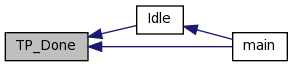UINT8 TP_GetAdXYOm ( UINT16 * x, UINT16 * y )

References CHX, CHY, start_touch(), TP_AD_Charge(), and TP_CS_L.

Referenced by Idle().

Here is the call graph for this function: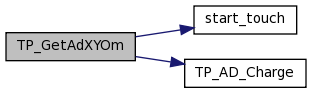Here is the caller graph for this function: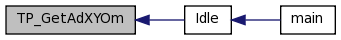void TP_Init ( )

References CS_PIN, DCLK_PIN, DIN_PIN, DOUT_PIN, and PINSEL1.

Referenced by Idle(), and main().

Here is the caller graph for this function:Generated on Wed Mar 23 11:08:35 2011 for Omnima LCD by1.6.3